# Histogram using Plotly in Python

• Last Updated : 28 Jul, 2020

Plotly is a Python library which is used to design graphs, especially interactive graphs. It can plot various graphs and charts like histogram, barplot, boxplot, spreadplot and many more. It is mainly used in data analysis as well as financial analysis. plotly is an interactive visualization library.

## Histogram in Plotly

A histogram is a graph where the data are stocked and the each stocked is counted and represented. More broadly, in plotly a histogram is an accumulated bar chart, with several possible accumulation functions. The data to be stocked can be numerical data but also categorical or date data. It is commonly used in dealing with large data of sets.

Syntax: plotly.express.histogram(data_frame=None, x=None, y=None, color=None, facet_row=None, facet_col=None, facet_col_wrap=0, hover_name=None, hover_data=None, animation_frame=None, animation_group=None, category_orders={}, labels={}, color_discrete_sequence=None, color_discrete_map={}, marginal=None, opacity=None, orientation=None, barmode=’relative’, barnorm=None, histnorm=None, log_x=False, log_y=False, range_x=None, range_y=None, histfunc=None, cumulative=None, nbins=None, title=None, template=None, width=None, height=None)

Parameters:
data_frame: DataFrame or array-like needs to be passed for column names
x: Either a name of a column in data_frame, or a pandas Series or array_like object. Values from this column or array_like are used to position marks along the x axis in cartesian coordinates.
y: Either a name of a column in data_frame, or a pandas Series or array_like object. Values from this column or array_like are used to position marks along the y axis in cartesian coordinates.
color: Either a name of a column in data_frame, or a pandas Series or array_like object.

Example: Using the tips Dataset

## Python3

 `import` `plotly.express as px`` ` `df ``=` `px.data.tips()`` ` `fig ``=` `px.histogram(df, x``=``"total_bill"``)``fig.show()`

Output: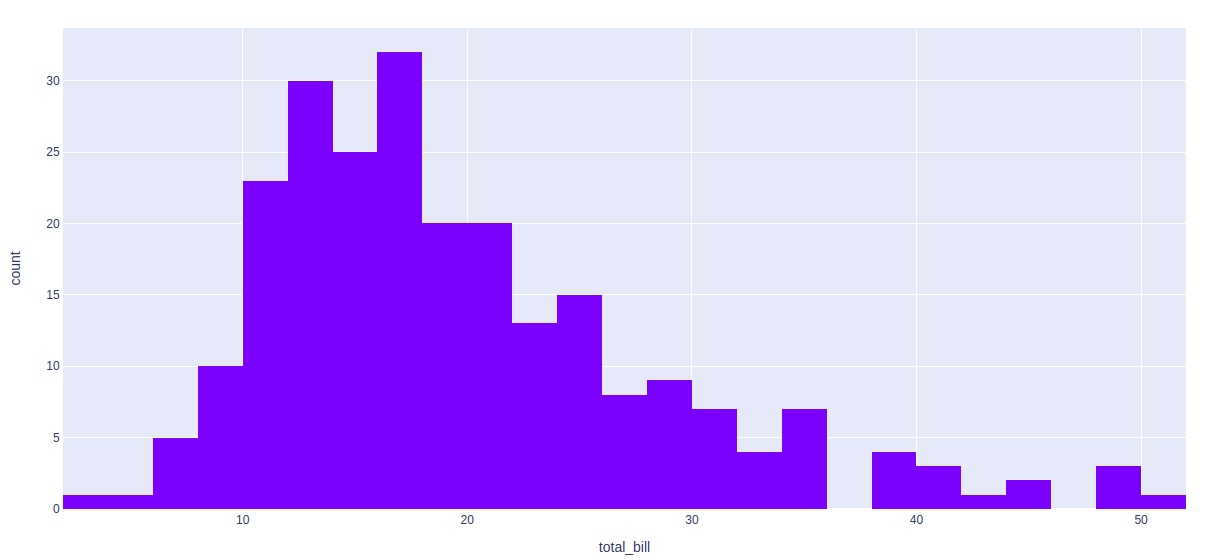## Type of normalization

By default, mode through which the bins are represented is count of samples. We can change this mode using Plotly. Ir=t can be done using histnorm argument. Different values that can be passed using this argument are-

• percent or probability: The output of histfunc for a given bin is divided by the sum of the output of histfunc for all bins.
• density: The output of histfunc for a given bin is divided by the size of the bin.
• probability density: The output of histfunc for a given bin is normalized such that it corresponds to the probability that a random

Example 1:

## Python3

 `import` `plotly.express as px`` ` `df ``=` `px.data.tips()`` ` `fig ``=` `px.histogram(df, x``=``"total_bill"``,``                   ``histnorm``=``'probability density'``)``fig.show()`

Output: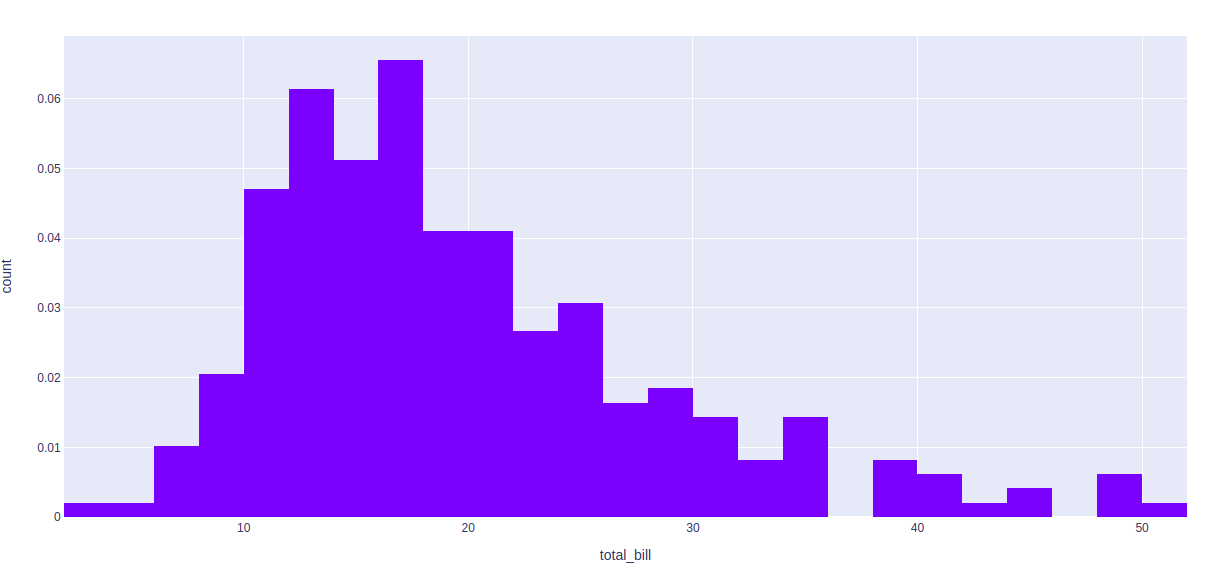Example 2:

## Python3

 `import` `plotly.express as px`` ` `df ``=` `px.data.tips()`` ` `fig ``=` `px.histogram(df, x``=``"total_bill"``, histnorm``=``'percent'``)``fig.show()`

Output: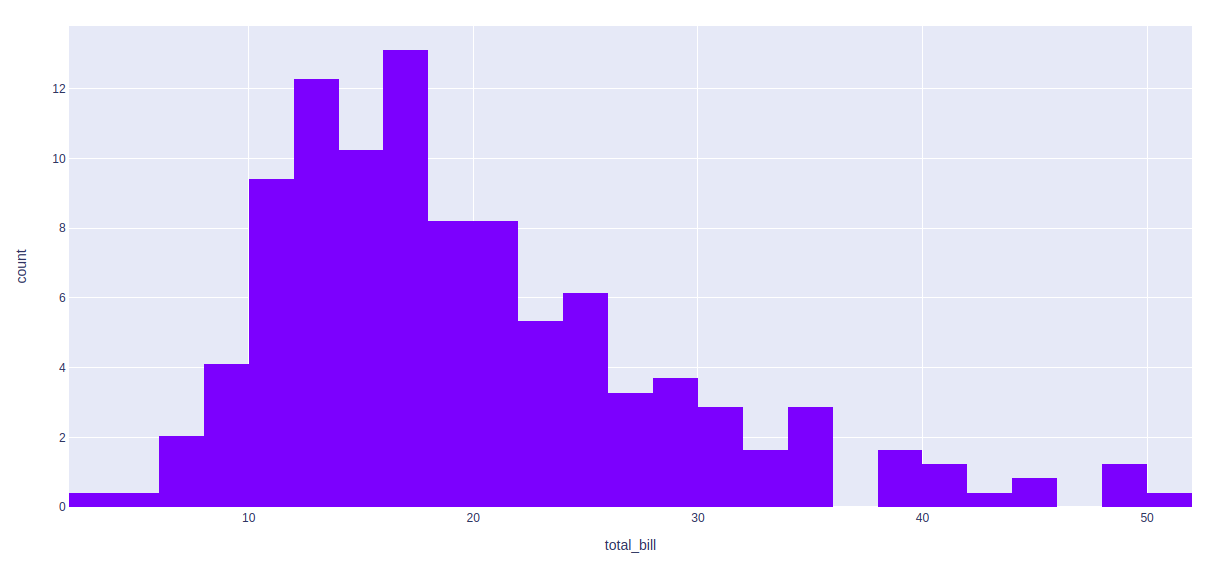## Selecting the number of bins

By default, the number of bins is chosen in such a way that this number is comparable to the typical number of samples in a bin. In plotly  the number, as well as range of values, can be customized by using nbins parameter.

Example:

## Python3

 `import` `plotly.express as px`` ` `df ``=` `px.data.tips()`` ` `fig ``=` `px.histogram(df, x``=``"total_bill"``, ``                   ``histnorm``=``'percent'``, ``                   ``nbins ``=` `10``)``fig.show()`

Output: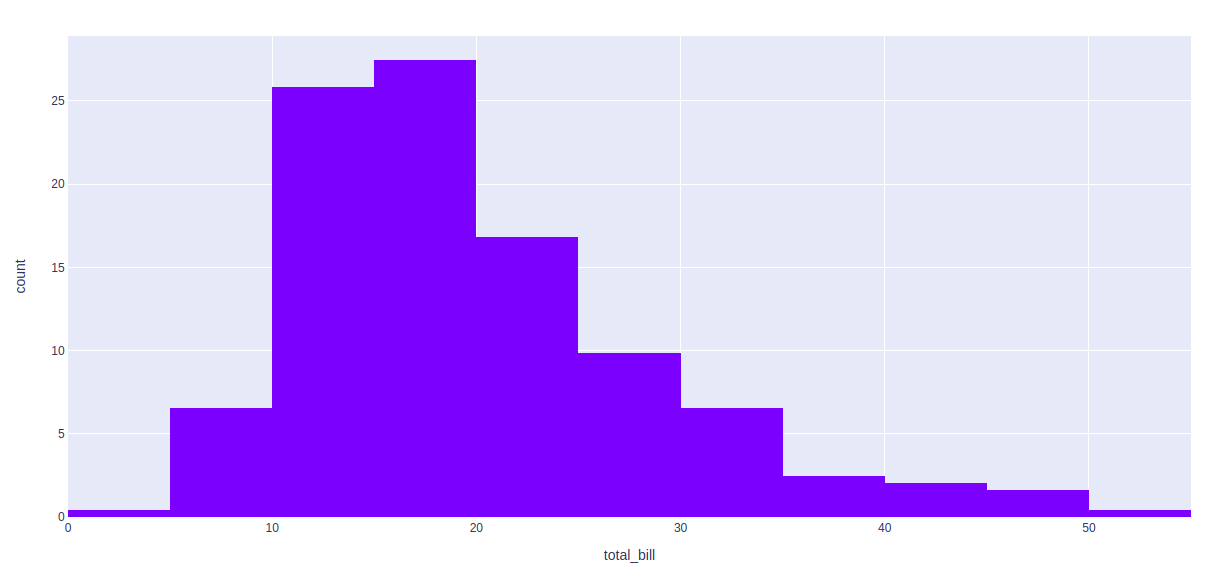## Stacked values in Histogram

In plotly different values can be shown in one column by using the color argument.

Example:

## Python3

 `import` `plotly.express as px`` ` `df ``=` `px.data.tips()`` ` `fig ``=` `px.histogram(df, x``=``"total_bill"``, color ``=` `"smoker"``)``fig.show()`

Output: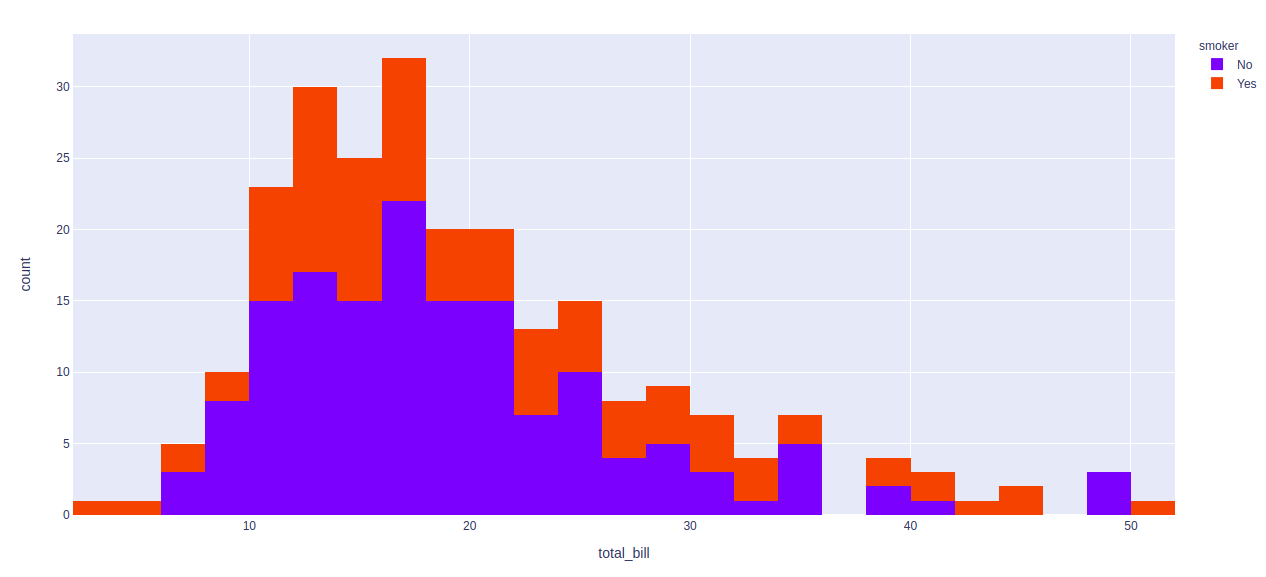## Visualizing the underlying distribution

In plotly, by using the marginal parameter we can visualize the distribution of the values. Marginal parameter has three values:

• rug
• box
• violin

Example:

## Python3

 `import` `plotly.express as px`` ` `df ``=` `px.data.tips()`` ` `fig ``=` `px.histogram(df, x``=``"total_bill"``, marginal ``=` `'box'``)``fig.show()`

Output: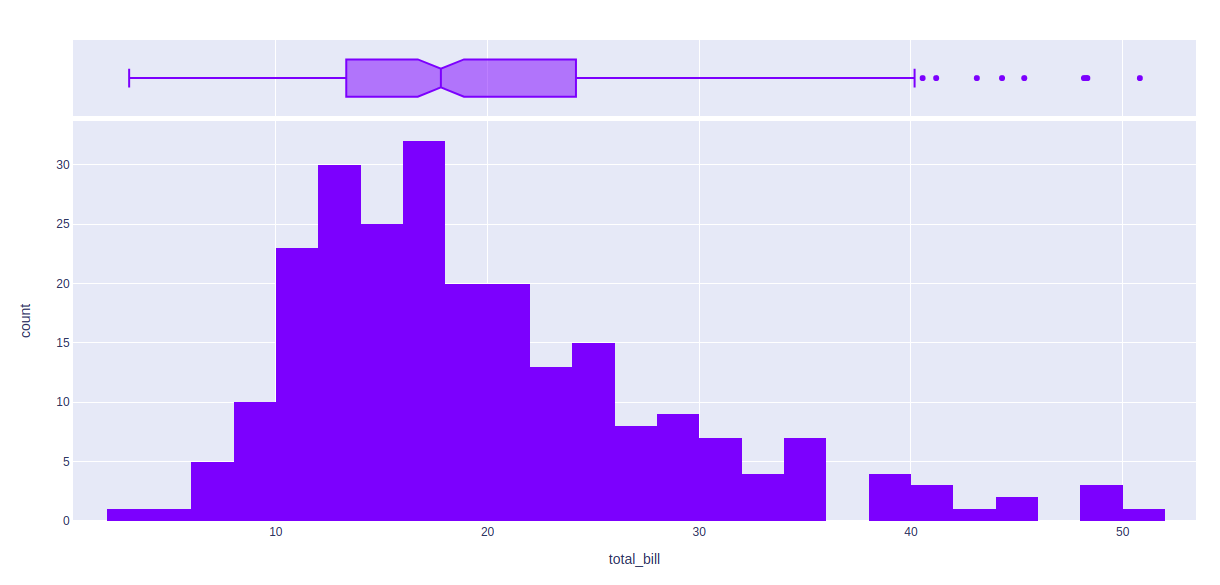My Personal Notes arrow_drop_up